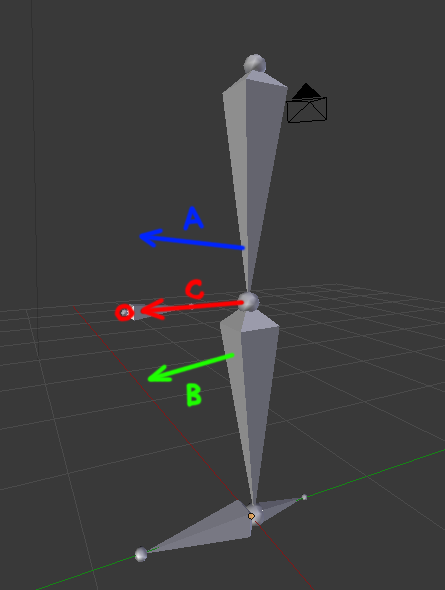# How to set the position of IK Pole Target using script?

Hello all! I am working on a script for FK to IK switching. The main problem in this case seems to be updating the position of IK pole target bone based on FK poseMy presumed algorithm was: calculate the “forward” vectors for first and second bones in a chain (vectors A and B), then interpolating between A and B (mathutils.Vector.slerp) to get offset vector ©, and finding the final position of pole target as a sum of vector C and the position of the second bone in a chain. But when I try to set the resulting position in Pose mode (using PoseBone.location), the result is nowhere near the expected position. Either I calculate the directional vectors wrong (I multiply the bones’ rotation quaternions to vectors) or the way I set the bone position is wrong (PoseBone.location) Which is it? The code is the following:

``````import mathutils
import math
import bpy

this = bpy.data.objects["Armature"]
pole = this.pose.bones["ikHint"]
thigh = this.pose.bones["thigh"]
calf = this.pose.bones["calf"]

thighDir = thigh.rotation_quaternion * mathutils.Vector((1.0,-0.0,0.0))
calfDir = calf.rotation_quaternion * mathutils.Vector((1.0,-0.0,0.0))
jointDir = mathutils.Vector.slerp(thighDir,calfDir,0.5).normalized()

pole.location =  calf.location + jointDir
``````

I recently tried to do it the easier way, without (or with minimum) scripting, but ran into the problem as well.
My plan was to parent 2 dummies A and B to thigh and calf correspondingly, with some forward offset, and make the 3rd dummy follow their averaged position using constraints. That would be the expected IK pole target position. But what constraints should I use? Two Copy Location constraints, with 0.5 weight each, do not give expected result
https://gyazo.com/dee01240cd75e7371f72489b71a34bab

Ahh I see what’s going on.

So… PoleDummy C starts at it’s origonal possision - the possision you set in object mode.

Then it’s position is mixed 50%/50% with the position of A - so it’s 50% it’s original position, and 50% A’s position.

Then it’s position is mixed 50%/50% with the position of B - so it’s 25% it’s original position, 25% A’s position, and 50% B’s position.

So I have to set the 1st constraint to 1.0 and another to 0.5?